# The Importance of Learning about Proportions in Math

## What are proportions?

Proportions are a fundamental concept in mathematics that help us compare the relative sizes or quantities of two or more things.

## Understanding ratios

Before we dive into proportions, it's important to understand ratios. A ratio is a comparison of two quantities. It's typically written in one of three ways:

As a fraction: For example, the ratio of apples to oranges can be written as "3/2," which means there are 3 apples for every 2 oranges.

Using a colon: The same ratio of apples to oranges can be written as "3:2."

Using the word "to": You can also express it as "3 to 2."

## Proportions defined

A proportion is essentially an equation that states that two ratios are equal. In other words, if you have two sets of numbers in a proportion, the cross products of those ratios are equal.

## The basic proportion formula

The simplest form of a proportion looks like this: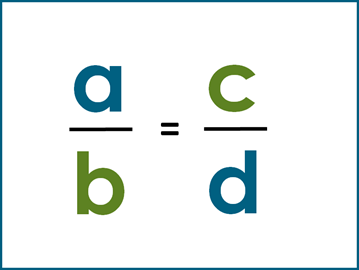In this equation, a and d are called the extremes, while b and c called the means. The key idea here is that the product of the extremes (a×d) is equal to the product of the means (b×c).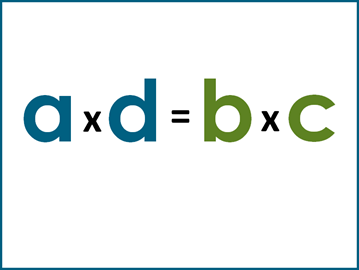## Solving proportions

To solve a proportion, you can use the basic proportion formula and cross-multiply. Here are the steps:

Cross-multiply: Multiply the values diagonally across the equal sign.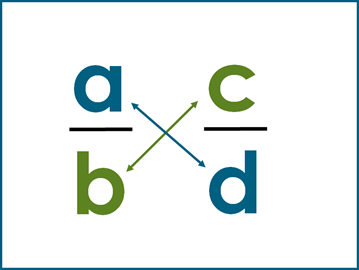a × d and b × c.

Solve for the missing value by isolating it on one side of the equation.

For example, if you're trying to find a, divide both sides by d.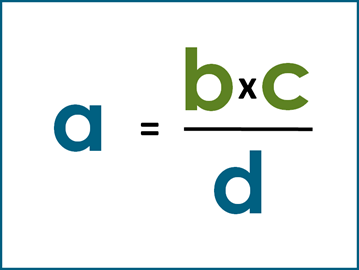## Proportions example

Let's say you have a proportion:  2 / 3 = a / 6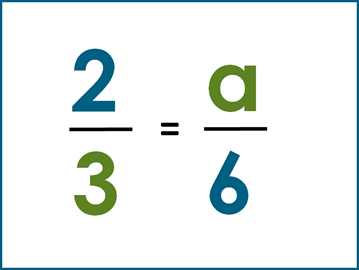To solve for a, you would cross-multiply: 2 × 6 = 3 × a.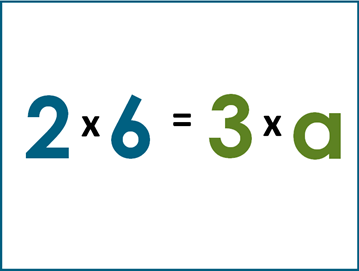Then, simplify: 12 = 3 x a

Finally, isolate a by dividing both sides by 3: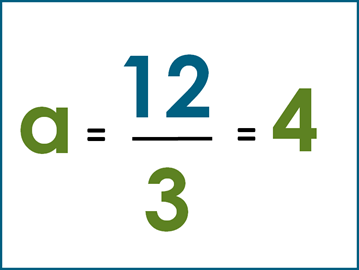That's the basic idea of simple proportions in math. They are incredibly useful for solving problems involving ratios and comparing quantities in real-world scenarios.

## Proportions worksheets

To help students practice proportions in math, we’ve created several worksheets covering simple proportions as well as proportions word problems.

## Simple proportions worksheets

In our grade 6 math worksheets section we have a set of worksheets for students to practice simple proportions.## Practice proportions word problems

To think about real-world scenarios, we have also created word problems worksheets for practicing proportions.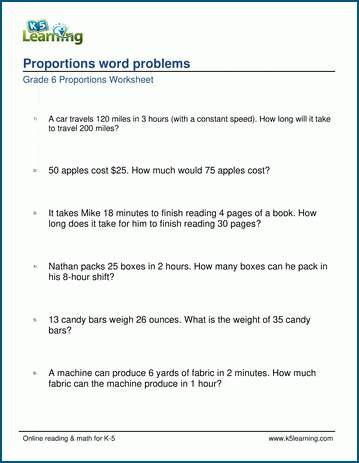Become a Member

This content is available to members only.